Nanoelectromechanical System (NEMS): Observing Mechanical Nonlinearity

Seung Sae Hong October 31, 2007

(Submitted as coursework for Physics 210, Stanford University, Autumn 2007)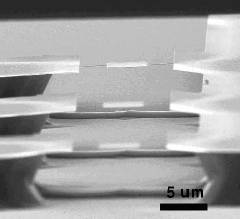Fig. 1: Torsional resonator structure of Pd metal thin film. Scanning Electron Microscope picture from Hong et al..

Nanoelectromechanical System (NEMS) is a mechanical structure characterized by extremely small dimension of nanometer scale. Microelectromechanical system (MEMS) has been successively used in many applications such as micromachines, fluid controlers and acceleration sensors. In the series of achievements, the tiny mechanical structure keeps shrinking its size, which enables demonstrations of on-chip physics. By relatively simple methods of semiconductor fabrication processes, scientists build a physical system with reduced mass and increased resonant frequency. From mesoscopic electron transport to magnetic nanoscale devices, NEMS broadens it's realm to fundamental science rather than commercial electronics.[3,4]

The advantages of NEMS - small size and mass - offer the chance to actuate the structure enough to observe the mechanical nonlinearity in a laboratory circumstance. The most simple mechanical structure is a suspended beam; but in order to see both translational and torsional motions of the structure, physicsists contrieved the structure named 'torsional resonator' - a rectangular paddle suspended by two thin beam. Using the simple equations of mechanics and material science, we can pull out the nonlinearity phenomena originated from the tensile stress in motion.

Fabrication of NEMS Device

The fabrication scheme is illustrated in Fig.2. In general, the substrate for device processing consists of heterostructures (CVD) techniques. The common system to realize the structure are Si/Silicon Oxide/Si and GaAs/InGaP/GaAs.[6,7] The top layer become the structure itself where the second layer is removed to suspend the structure. Adopting standard lithography techniques using electron beam or Ultra-violet lay, the planar shape of the structre is patterned on the sample. After seletively devloped the resist layer, we can evaporate metal layer to protect the pattened shape.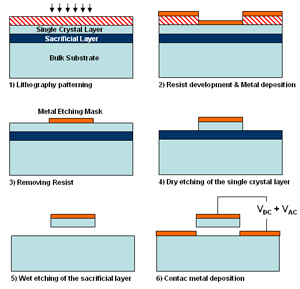Fig. 2: Fabrication steps to realize the device.

The torsional resonator is formed through two etching process - dry etching and wet etching. By the exposure to aculeated reactive ions, unnecessary part of the crystal layer is etched vertically while the metal mask preserves part below the resonator patten. After that, chemical reaction with acid/base makes the sacrificial layer be removed so that the whole structure is suspended. Metal layer is deposited once again in order to form a electrical contact to apply driving voltage for the actuation. From this relatively simple method, scientist can make the extremely small structure of several tens nanometer, both in lateral and planar dimension.

Actuation of the Structure to Mechanical Modes

To introduce the motion of small structure, applying force and external energy is required. The structure is actuated and starts to vibrate when we applying force of which frequency is coherent with the resonant frequency. That is, we should supply AC signal to the structure to inducing it's vibrational modes. The easiest method is to actuate the resonator electrostatically. The voltage between the structure's metal layer and bottom electrodes can be regarded as a capasitor system and Coulomb force between the plate is the source of actuation. Electrostatic driving method can induce large force to the structure easily - but it's disadvatageous to measure the driving force accurately. Another useful method is the magnetomotive driving, which use the Lorentz's law to induce the motion. In the transverse magnetic field, the alternating current along the resonator's axis experiences the Lorentz's force. We can calculate the force easily - from the current density and magnetic field - but the usage in magnetic system is limited.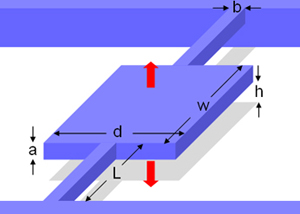Fig. 3: Dimension of a torsional resonator. The red arrows indicate the fundamental translational mode which the central paddle moves up and down.

Detecting the movement of such small object is also a chanllenging part. To catch a tiny displacement of the structure(~augstrom), physicists use optical interferrometer first. By placing the focal point of the laser beam, we can measure the displacement information by detecting changes in reflected beam into a photodetector. Detecting turneling current in small gap and measurement of the capacitance between the structure and the bottom electrode are alternative methods used frequently, depending on the structure's conditions.

From the equations of continuum mechanics, we can predict the vibrational modes of the structure. Intuitibly, the fundamental mode - of the lowest resonant frequency - is either the torsional mode or the translational mode of no node. Torsional motion's resonance is related with the Shear modulus of the structure while the translational resonant frequency is proportional to the square root of it's dynamic Young's modulus. In case of the typical dimensions of Si-based NEMS resonator, the first resonant frequency is an order of Radio Frequency (RF), about 1MHz ~ 100MHz.

Characterization of Nonlinearity in Translational Motion

From the analysis of the first translational mode, we expect to see the "Duffing Oscillator," which is a deviation from a simple harmonic oscillator due to a nonlinear cubic term. In this calcuation, the actuating force is regarded as the electrostatic force between the metal plates. Using the geometric dimensions from Fig.3, the analytic solution of the motion can be induced. To apply the mechanical equations to the torsional resonator structure, we assume two postulates; 1) The vibration of the fundamental translational mode is mainly due to the flexure of 'relatively thin' beams suspending the paddle. 2) The boundary condition of the beam is 'clamped', which means the edge displacement δedge = 0. In general, we can describe the translational motion with the equation of a damped oscillator with external force F(ωt). The equation is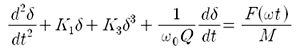where δ is a displacement of vertical direction and ω0 is the resonant frequency. K1 and K3 represent a spring constant and a cubic constant each where M is the total mass of central paddle and Q means Q-factor of the structure. We can define the spring constant by evaluating the linear restoring force Fz.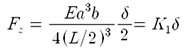E is the dynamic Young's modulus of the material. In addition, we can characterize the cubic term, which brings the nonlinearity into the system. The K3 is from the beam stretching, where ε means the strain in the beam and φ indicates the beam's angular displacement.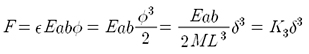And we can simply determine the force term by deferentiating electrostatic energy. To induce the cubic term from the beam stretching, we need DC bias to form the static flexure in beams, by pulling down the structure. Therefore, the voltage term consists of both AC signal and DC voltage. The original equation of motion becomes below equation.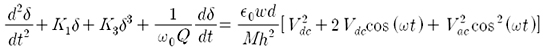The solution of the equation of motion is of the form below.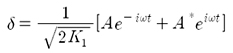The differential equation is simplified as the next cubic equation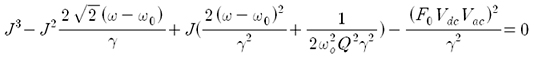where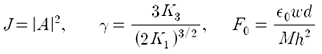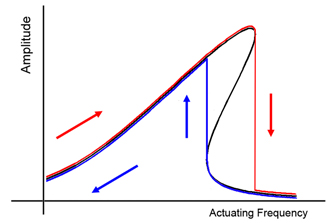Fig. 4: A brief sketch of the theoretical solution and experimental observation of resonant peak of Duffing Oscillator. Red line means up-sweep of frequency and blue line indicates down-sweep graph where black line is a mathmatical solution of Duffing equation.

The cubic equation has either one real solution or three real roots depending on the constants. If the equation has three real solutions, it means the J-ω graph is not a single valued function, which shows the hysteretic shape near the resonant peak as shown in Fig.4. In the experimental system, we can change the constant by applying different AC and DC voltage. With the existance of the DC bias(=5V), the frequncy-swept measurement indicates that the sudden step down of resonace peak appears as small increase in AC modulation voltage(~10mV). From the experiment, we can observe the onset of nonlinearity in mechanical vibration within an augstrom scale, which is originated from the Duffing equation.

© 2007 Seung Sae Hong. The author grants permission to copy, distribute and display this work in unaltered form, with attribution to the author, for noncommercial purposes only. All other rights, including commercial rights, are reserved to the author.

References

 H. G. Craighead, Science 290, 1532 (2000).

 K. L. Ekinci and M. L. Roukes, Rev. Sci. Instrum. 76, 061101 (2005).

 D. W. Carr et al., Appl. Phys. Lett., 77, 1545 (2000).

 A. N. Cleland and M. L. Roukes, Nature 392, 160 (1998).

 S. S. Hong et al., Proc. of Mat. Res. Soc. 1018E (2007).

 D. W. Carr and H. G. Craighead, J. Vac. Sci. Technol. B, 15, 2760 (1997).

 S. B. Shim et al., Appl. Phys. Lett. 91, 133505 (2007).

 S. Evoy et al., J. Appl. Phys. 86, 6072 (1999).

 A. N. Cleland and M. L. Roukes, Appl. Phys. Lett. 69, 2653 (1996).

 J. A. Walker, K. W. Goossen and S.C. Arney, J. Microelectromech. Syst. 5, 45 (1996).

 J. B. Marion and S. T. Thornton, Classical Dynamics of Particles and Systems(4th Ed.) (Saunders College Publishing, Fort Worth, 1995), p.168.